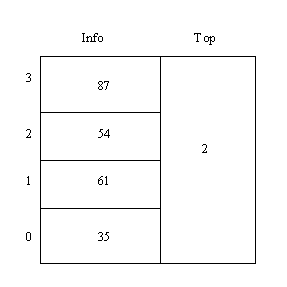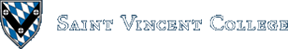Computing & Information Systems    DepartmentSearch CIS Site      Tutorials

## Software Design Using C++

### What is a Stack?

Intuitively, a stack is like a pile of plates where we can only remove a plate from the top and can only add a new plate on the top. In computer science we commonly place numbers on a stack, or perhaps place records on the stack. The following snippet of code from lstclass.h shows the typical stack operations that we plan to use (other than the automatically available constructor and destructor).

 `````` bool Empty(void) const; void Push(const ItemType & Item); void Pop(ItemType & Item); ``````

The `Push` operation places a new data item on top of the stack. `Pop` removes the top item and sends it back via the parameter. (Sometimes Pop is implemented so that the removed item is returned in the function name instead.) Finally, the `Empty` operation tells us whether or not the stack object is empty. Here is a picture showing a small stack of integers as well as the same stack after 34 has been pushed: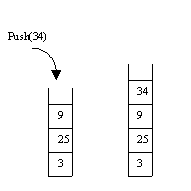A stack is called a "last in, first out" (LIFO) data structure since the last item pushed onto a stack will be the first one popped off. It can also be called a "first in, last out" (FILO) data structure since the first item pushed onto it will be the last one popped off. Because of these features, a stack is the ideal data structure to use in reversing a sequence of items. A section of code for doing this follows:

 `````` cout << "Enter a number (CTRL z to end): "; cin >> Number; while (! cin.fail()) { Stack.Push(Number); cout << "Enter another number (CTRL z to end): "; cin >> Number; } cout << endl << endl << "Numbers in reverse order are:" << endl; while (! Stack.Empty()) { Stack.Pop(Item); cout << Item << endl; } ``````

Note how simple this algorithm is! One pushes the numbers one by one onto the stack. Then one pops numbers off, printing each number popped, until the stack is empty.

Since our picture of a stack essentially shows a sequence of data items, it should be easy to hold the data for a stack in a linked list. After all, we would only need to directly access the data at one end of the list, the front, which would represent the top of the stack.

The group of files given below contains a linked-list implementation of a class called LstStackClass as well as a test program that reverses a sequence of numbers using the algorithm just examined. The first file sets up the type name `ItemType` for whatever kind of data we want to place on our stacks. The next four files are almost the same ones that we used in the section on lists to create a class called `ListClass`. The only significant change is to the GetNode function in list.cpp. This function has been changed to use new inside of a `try...catch` when it tries to allocate a new node, whereas we formerly used the nothrow option to return a `NULL` pointer if the new operation failed to allocate the desired memory space. The use of the `try...catch` is a more modern way to handle the failure to allocate memory. The last file contains the test program itself. So, what about the files lststack.h and lststack.cpp? The former contains the class declaration for `LstStackClass`, which is repeated here for convenience:

 `````` #include "list.h" class LstStackClass { public: // No constructor is mentioned here. We instead use the default // constructor automatically supplied by the compiler. bool Empty(void) const; void Push(const ItemType & Item); void Pop(ItemType & Item); private: ListClass List; // an embedded List object }; ``````

There is only one data field, and it is private, as usual. This field contains a `ListClass` object. In other words, each stack object will contain an embedded list object to hold the data. Thus we have to include the `list.h` header file in order to have access to the `ListClass` declaration.

The lststack.cpp file contains the code for our stack operations. This is really easy, because all of the work is done by using the appropriate list functions, which have already been implemented. Thus, the function to see if a stack is empty just checks if the embedded list is empty. Similarly, to push a data item onto the stack, we just insert it at the front of the list as follows:

 `````` void LstStackClass::Push(const ItemType & Item) { List.InsertFront(Item); } ``````

Finally, the pop operation is carried out by removing the front data item from the embedded list. Look though all of the source code files given above for the complete details on this example. Note that our `LstStackClass` only provides for stacks that hold items of one particular type. Such a class would not allow us to place a stack of integers and a stack of characters into the same program, for example. There is a way in C++ to create a more generic stack type that would let us create such different stacks within the same program. This method involves the use of templates and is beyond the scope of what can be covered at this point.

### Alternative Stack Implementation

This implementation also uses an embedded linked list, but derives the LstStackClass from the following abstract base class. This abstract base class shows the typical stack operations that we plan to use (other than the automatically available constructor and destructor). An abstract base class cannot be used for the creation of objects. Rather, it is just an outline of sorts, from which we derive by inheritance a class that actually implements the operations and with which we can create objects.

 `````` class StackBaseClass { public: virtual void Push(const ItemType & Item) = 0; virtual void Pop(ItemType & Item) = 0; virtual bool Empty(void) const = 0; }; ``````

The term `virtual` indicates that these are virtual functions, the type of functions that support dynamic (run-time) binding. Without the word `virtual` static binding would be used. With static binding, all function calls have to be figured out at compile time. It may be that we will never need dynamic binding in the type of programs that we will write, but it is safer to allow for that possibility.

The `= 0` at the end of each function indicates that these are pure virtual functions, functions with no code attached. A base class containing at least one pure virtual function is called an abstract base class. Since there is no code (for at least one of the class functions), objects of such a class cannot be created.

The group of files given below contains a linked-list implementation of a class called LstStackClass as well as a test program that reverses a sequence of numbers, much like we did with our previous stack implementation. The first file sets up the type name `ItemType` for whatever kind of data we want to place on our stacks. The next four files are the same ones that we used in the section on lists to create a class called `ListClass`. The stack.h file contains the abstract base class outlined above that specifies the three main list operations that we want in any stack implementation. The last file contains the test program itself.

Look more carefully at the files lststack.h and lststack.cpp. The former contains the class declaration for `LstStackClass`, which is repeated here for convenience:

 `````` #include "stack.h" #include "list.h" class LstStackClass: public StackBaseClass { public: // No constructor is mentioned here. We instead use the default // constructor automatically supplied by the compiler. bool Empty(void) const; void Push(const ItemType & Item); void Pop(ItemType & Item); private: ListClass List; // an embedded List object }; ``````

Note that the new class is derived by public inheritance from ``` StackBaseClass```, our abstract base class. Therefore, we must include the `stack.h` file that contains the declaration of this abstract base class.

There is only one data field, and it is private, as usual. This field contains a `ListClass` object. In other words, each stack object will contain an embedded list object to hold the data. Thus we have to include the `list.h` header file in order to have access to the `ListClass` declaration.

The lststack.cpp file contains the code for our stack operations. This works the same way as in our previous implementation of a stack.

If you look through the files that comprise this implementation of a stack, the only real difference is that LstStackClass is derived by public inheritance from an abstract base class. Everything else is the same.

### Evaluating a Postfix Expression

You may be asking what a stack is good for, other than reversing a sequence of data items. One common application is to convert an infix expression to postfix. Another is to find the value of a postfix expression. We will not look at the conversion algorithm here, but we will examine the algorithm to evaluate a postfix expression.

First, let's explain the terminology. An infix expression is the type that we are used to in ordinary algebra, such as `3 + 9`, which is an expression representing the sum of 3 and 9. Infix expressions place their (binary) operators between the two values to which they apply. In the above example, the addition operator was placed between the 3 and the 9.

A postfix expression, in contrast, places each operator after the two values to which it applies. (Post means "after", right?) The above expression would be `3 9 +`, when rewritten in postfix.

Here are a few more examples in the following table. The infix form is shown on the left, and the postfix form is given on the right.

Infix: Postfix:
16 / 2 16 2 /
(2 + 14) * 5 2 14 + 5 *
2 + 14 * 5 2 14 5 * +
(6 - 2) * (5 + 4) 6 2 - 5 4 + *

Note that postfix expressions do not use parentheses for grouping; parentheses are not needed! Infix sometimes requires parentheses to force a certain order of evaluation. For example, in the second example above, parentheses were needed to indicate that the addition should be done before the multiplication. Without the parentheses you get the third example, where the multiplication is done before the addition (using the precedence rules from ordinary arithmetic, or from C++, for that matter).

Arithmetic expressions like the above can of course be much longer. We could also allow other operators, such as ^ for exponentiation, or perhaps a unary minus. A sample infix expression that uses exponentiation is `4 ^ 2`, which means 4 to the second power. A unary minus is sometimes used as in the infix expression -(4 + 2). A unary operator is one that is applied to a single value, as opposed to the typical binary operators, which are applied to two values. We will not consider unary operators or exponentiation further here.

The algorithm to evaluate a postfix expression works like this: Start with an empty stack of floats. Scan the postfix expression from left to right. Whenever you reach a number, push it onto the stack. Whenever you reach an operator (call it Op perhaps), pop two items, say `First` and `Second`, and then push the value obtained using `Second Op First`. When you reach the end of the postfix expression, pop a value from the stack. That value should be the correct answer, and the stack should now be empty. (If the stack is not empty, the expression was not a correct postfix expression.)

Let's look at the postfix expression evaluation algorithm by way of example. Consider the postfix expression `2 14 + 5 *` that was mentioned above. We already know from its infix form, `(2 + 14) * 5`, that the value should be `16 * 5 = 80`. The following sequence of pictures depicts the operation of the algorithm on this example. Read through the pictures from left to right.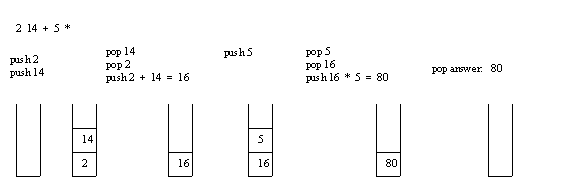Let's evaluate another postfix expression, say `2 10 + 9 6 - /`, which is `(2 + 10) / (9 - 6)` in infix. Clearly the value should work out to be `12 / 3 = 4`. Trace through the algorithm by reading the following pictures from left to right.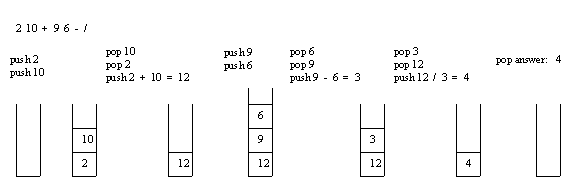When one reaches an operator in this algorithm, it is important to get the order right for the values to which it applies. The second item popped off should go in front of the operator, while the first one popped off goes after the operator. You can easily see that with subtraction and division the order does matter.

A good exercise for the reader is to develop a program that repeatedly evaluates postfix expressions. In fact, with enough work, it can be turned into a reasonable postfix calculator.

### An Array Implementation

A stack can also be implemented using an array-based approach. Look at the example contained in the following list of files. As in our second list-based implementation, a new class is derived from the abstract base class named `StackBaseClass`. However, this time, instead of using an embedded list object, the data fields consist on an integer `Top` and an array `Info`.
 itemtype.h Header file for setting up ItemType. stack.h Header file for the abstract base class. arrstack.h Header file for array-based stacks. arrstack.cpp Code file for array-based stacks. reverse.cpp Test program (number reversal).

The main idea is that `Top` holds the index of the top of stack item, which is stored in the `Info` array. To push a new item onto a stack, `Top` is simply incremented and the new item placed into the array at that index. To pop an item from the stack, the item at index `Top` is sent back and `Top` itself is decremented. Since a value of zero in `Top` indicates a stack with one item (at index 0), a value of -1 in `Top` indicates an empty stack.

The following drawing shows you a picture of an array-based stack with 3 items in it. Note that a `Top` value of 2 means that the top of stack item is at index 2 (and that the count of the number of items in the stack is one bigger, namely 3). The data item 87 at index 4 is simply garbage data. Thus this stack contains 35, 61, and 54, with the 54 at the top of the stack. The array would typically be a lot bigger than an array of 4 items; the small size was used so that the picture could be easily drawn.